Courses

# Structural Dynamics Civil Engineering (CE) Notes | EduRev

## Civil Engineering (CE) : Structural Dynamics Civil Engineering (CE) Notes | EduRev

The document Structural Dynamics Civil Engineering (CE) Notes | EduRev is a part of the Civil Engineering (CE) Course Topic wise GATE Past Year Papers for Civil Engineering.
All you need of Civil Engineering (CE) at this link: Civil Engineering (CE)

Q.1 A simple mass-spring oscillatory system consists of a mass m, suspended from a spring of stiffness k. Considering z as the displacement of the system at any time f, the equation of motion for the free vibration of the system is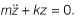The natural frequency of the system is    [2019 : 1 Mark, Set-I]
(a) m/k
(b) k/m
(c)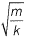(d)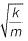Ans.
(D)
Solution: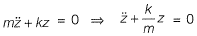Comparing with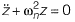We get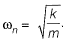Q.2 A plane frame shown in the figure (not to scale) has linear elastic springs at node H. The spring constants are kx = ky = 5 x 105 kN/m and kθ = 3 x 105 kNm/rad.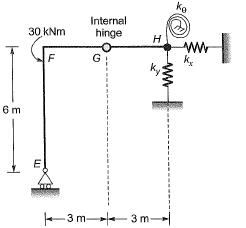For the externally applied moment of 30 kNm at node F, the rotation (in degrees, round off to 3 decimals) observed in the rotational spring at node H is___________    [2019 : 2 Marks, Set-II]
Solution: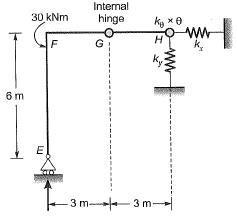No moment can be taken by segment FE
∴ MFE = 0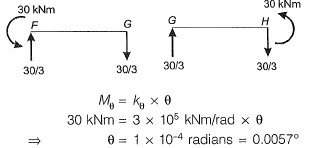Q.3 For the beam shown below, the stiffness coefficient K22 can be written as    [2015 : 1 Mark, Set-I]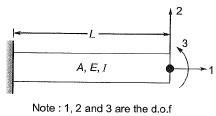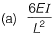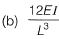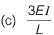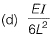Ans.
(B)
Solution: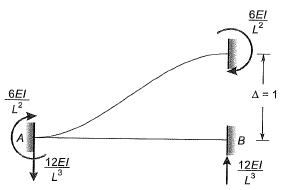By giving unit displacement in 2nd direction without giving displacement in any other direction, the force developed RB, is,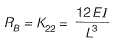(∴ Δ = 1)

Offer running on EduRev: Apply code STAYHOME200 to get INR 200 off on our premium plan EduRev Infinity!

,

,

,

,

,

,

,

,

,

,

,

,

,

,

,

,

,

,

,

,

,

;